The Library of Congress Classification System (LC)

AU Library, like most academic and research libraries, uses the Library of Congress (LC) Classification System, a classification system developed by the Library of Congress for its collection. All books on AU Library shelves are arranged according to the Library of Congress (LC) Classification System. The system is very useful for large academic libraries because of its capacity for generating and accommodating new subject headings. This system, which uses a combination of letters and numbers, ensures that books on similar subjects are shelved together (making it easier for browsing). This arrangement results in "serendipitous browsing:" you find one book in the catalog, go to the shelf, and, an even better book is sitting right next to it.

Tutorial (1)

Anatomy of a Library of Congress Call Number: LB2395 .F54 1989

Book title: Becoming a successful student

Author: Laraine E. Flemming

Call Number: LB2395. F54 1989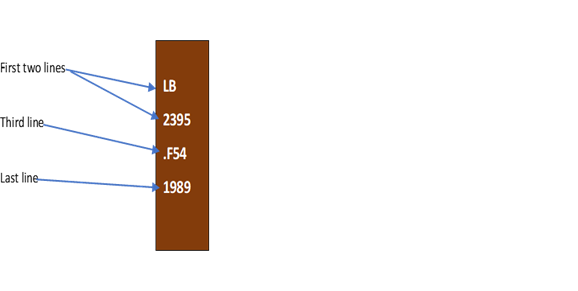Book title: Becoming a successful student

Author: Laraine E. Flemming

Call Number: LB2395. F54 1989

The first two lines describe the subject of the book.

LB2395 = Study skills - Reading (Higher education)

The third line often represents the author's last name.

F = Flemming

The last line represents the date of publication.

Tips for Finding Books on the Shelf: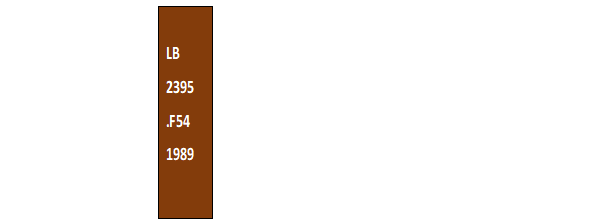Read call numbers line by line.

LB

Read the first line in alphabetical order:

A, B, BF, C, D... L, LA, LB, LC, M, ML...

2395

Read the second line as a whole number:

1, 2, 3, 45, 100, 101, 1000, 2000, 2430...

.F54

The third line is a combination of a letter and numbers. Read the letter alphabetically. Read the number as a decimal, e.g.:

.F54 = .54 .C724 = .724

Some call numbers have more than one combination letter-number line.

1989

The last line is the year the book was published. Read in chronological order:

1985, 1988, 1989, 1990, 1995...

Here is a shelf of books with the call number order explained: *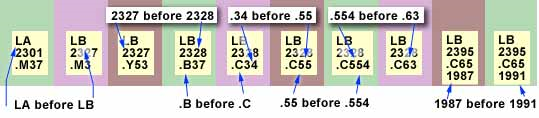* (Developed by University of Georgia).

Tutorial (2)

Materials are shelved alphabetically by the first line of the call number: *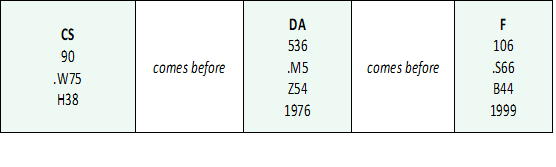If the first lines are the same, go to the next. This line is shelved in numerical order: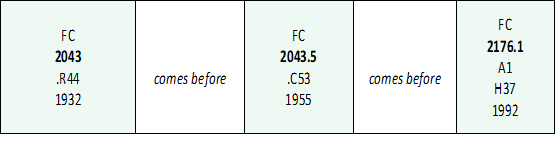If the first two lines are the same, then look at the next line. Here the arrangement is alphabetical, followed by decimal order. Some find it useful to add zeros to the decimal in order to create the same number of digits; then read them as you would whole numbers. (. G34 before. G50).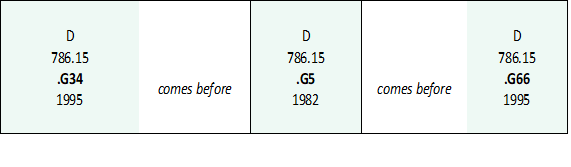* (Developed by University of Prince Edward Island).

Tutorial (3)

All of our materials are organized on the shelf using Library of Congress call numbers. When looking at the number on the book spine, these would be read one line at a time. *

For example:

DA
36
A77

Books are arranged in alphabetical order, by the letters on the first line of the call number. For example, first come all the D call numbers, then all the DA call numbers, then DB, etc.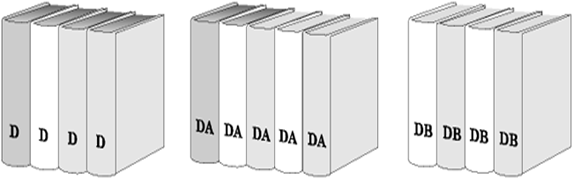Look at line 2

Within the DA call numbers, books are arranged in number order. The numbers are arranged in numerical order, from low to high.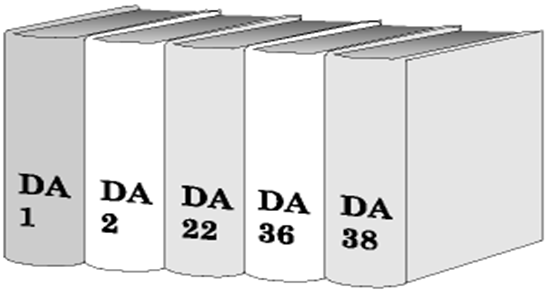Line 3

Line 3 of the call number has a letter and a number. The letters are in alphabetical order. Once you find the letter, read the number. But note these are decimals not whole numbers. Example: A55 is read as A .55. This is why A55 comes before A6 (A .55, A .6, A .65).

Note: Sometimes there will be a fourth Cutter number to further differentiate by author, title or subject or the year of publication. If there are multiple copies of the book, that may be indicated as well.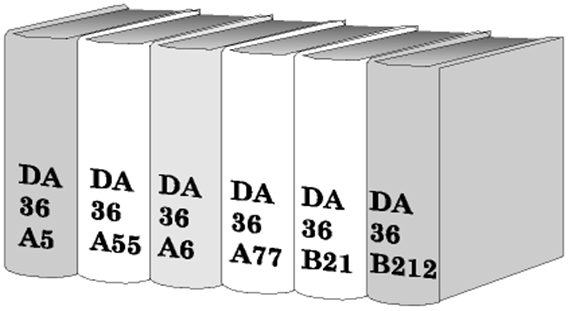* (Developed by University of Arizona).